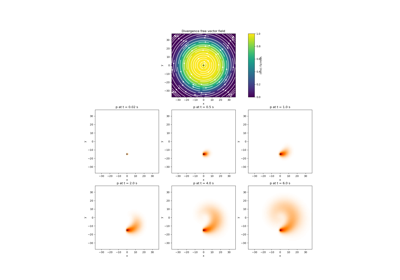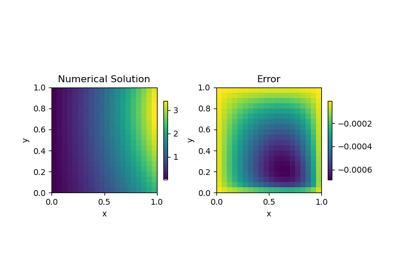# discretize.base.BaseRectangularMesh#class discretize.base.BaseRectangularMesh(shape_cells, origin=None, orientation=None, reference_system=None, **kwargs)[source]#

Base rectangular mesh class for the discretize package.

The BaseRectangularMesh class acts as an extension of the BaseRegularMesh classes with a regular structure.

Attributes

 nCx Number of cells in the x direction. nCy Number of cells in the y direction. nCz Number of cells in the z direction. nNx Number of nodes in the x-direction. nNy Number of nodes in the y-direction. nNz Number of nodes in the z-direction. n_cells Total number of cells in the mesh. n_edges_x Number of x-edges in the mesh. n_edges_y Number of y-edges in the mesh. n_edges_z Number of z-edges in the mesh. n_faces_x Number of x-faces in the mesh. n_faces_y Number of y-faces in the mesh. n_faces_z Number of z-faces in the mesh. n_nodes Total number of nodes in the mesh. shape_edges_x Number of x-edges along each axis direction. shape_edges_y Number of y-edges along each axis direction. shape_edges_z Number of z-edges along each axis direction. shape_faces_x Number of x-faces along each axis direction. shape_faces_y Number of y-faces along each axis direction. shape_faces_z Number of z-faces along each axis direction. shape_nodes The number of nodes along each axis direction.

Methods

 r(*args, **kwargs) r has been removed. reshape(x[, x_type, out_type, return_format]) Reshape tensor quantities.

## Galleries and Tutorials using discretize.base.BaseRectangularMesh#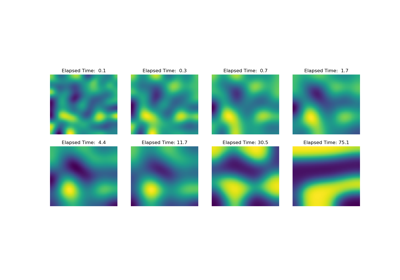Operators: Cahn Hilliard

Operators: Cahn Hilliard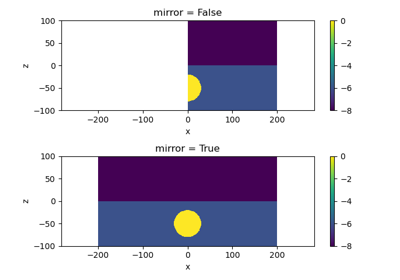Plot Mirrored Cylindrically Symmetric Model

Plot Mirrored Cylindrically Symmetric Model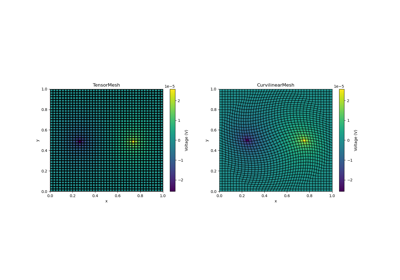Basic Forward 2D DC Resistivity

Basic Forward 2D DC ResistivityBasic: PlotImage

Basic: PlotImage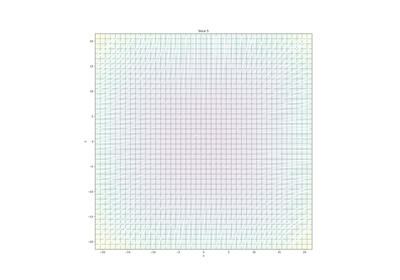Plotting: Streamline thickness

Plotting: Streamline thickness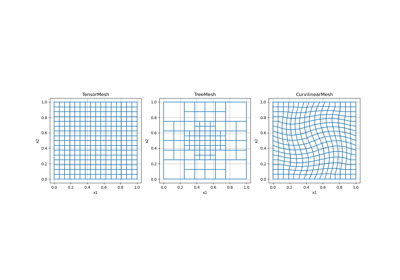Overview of Mesh Types

Overview of Mesh Types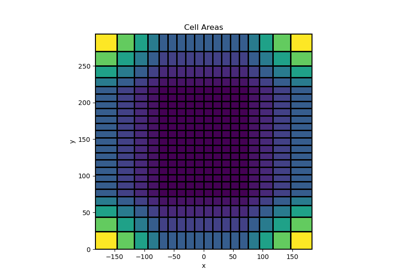Tensor meshes

Tensor meshes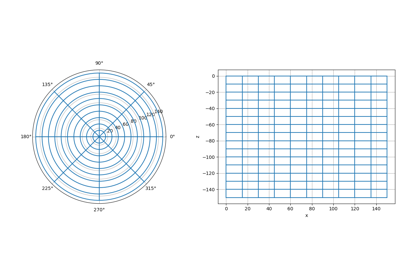Cylindrical meshes

Cylindrical meshes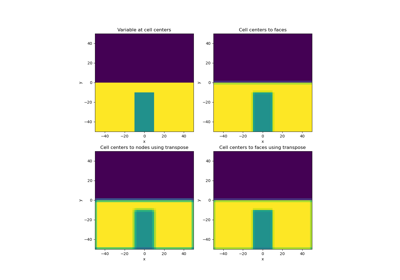Averaging Matricies

Averaging Matricies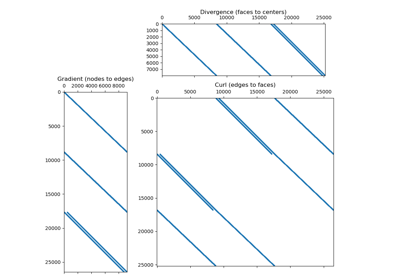Differential Operators

Differential Operators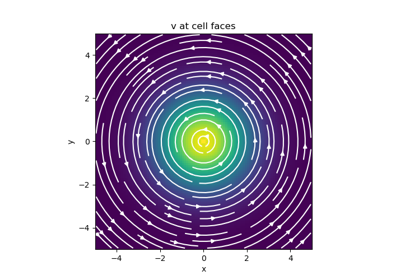Basic Inner Products

Basic Inner Products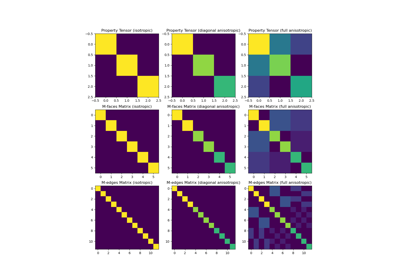Constitutive Relations

Constitutive Relations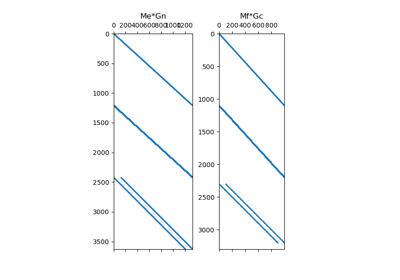Differential Operators

Differential Operators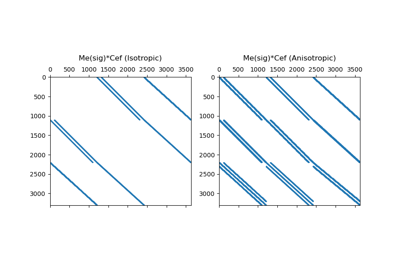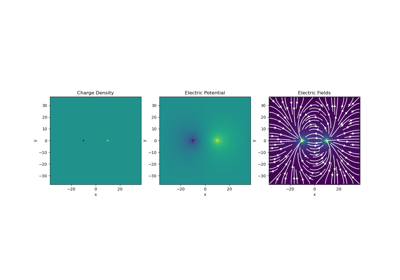Gauss’ Law of Electrostatics

Gauss' Law of Electrostatics